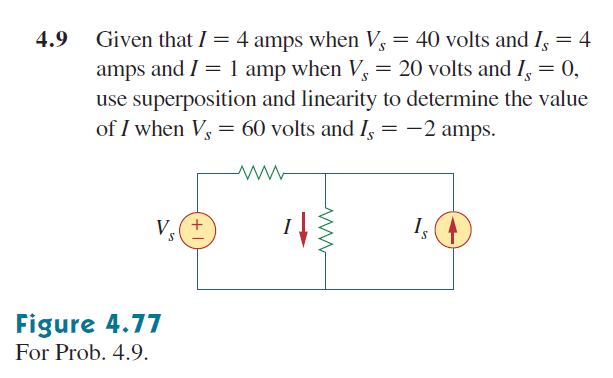# Using Superposition and Linearity to Find Current?

## Homework Statement## Homework Equations

Ohm's Law: v = iR
KVL: ∑ V = 0
KCL: ∑ I = 0

Linearity: kiR = kv

## The Attempt at a Solution

Okay, so to apply superposition, I'm supposed to turn off all independent sources except one. I began by turning off the voltage source, and I then I used KCL to attempt to find the voltage through the resistor with current I flowing through it. I named the resistor that is in series with the voltage source R1, and the resistor in parallel with the current source R2.

Is = (R1/(R1 + R2))Is + I

Then I tried to find the voltage attributed to the circuit by the current source:

v = ((R1/(R1 + R2))Is )R2

Then I turned off the current source, leaving an open circuit and tried to solve for the current from the voltage source:

vs = i(R1 + R2)

After this, I have tried plugging in the numbers given in the problem to find values for R1 and R2, but this didn't work out very well. I don't think I'm setting up or thinking about the problem correctly. Where have I gone wrong?

## Answers and Replies

mfb
Mentor
I don't see how you get your first equation.

It is possible, but you do not have to determine the resistance values. You can directly use superposition and linearity.

For example, if Is = 4 A and Vs = 40 V we know that I = 4 A.
What happens if Is = 2 A and Vs = 20 V?

How do we know that I = 4 A just from knowing that Is = 4 A and Vs = 40 V?

gneill
Mentor
If you assume linearity and superposition you can write the "output" as a linear equation of the "inputs". There are two inputs: a voltage source and a current source. There's one output: the current in the central branch. How might such a linear equation be written?

•Gwozdzilla
Okay, so just applying linearity... I made up the equation: I = mIs + nVs. Then I plugged in the given values and found that m = .5 and n = .05. This allowed me to get the correct answer to the problem when plugging in the final values. Does this have anything to do with superposition or was that just extraneous information from the initial question?

gneill
Mentor
Okay, so just applying linearity... I made up the equation: I = mIs + nVs. Then I plugged in the given values and found that m = .5 and n = .05. This allowed me to get the correct answer to the problem when plugging in the final values. Does this have anything to do with superposition or was that just extraneous information from the initial question?
Well, fundamentally superposition works because linearity does for circuits with linear components. It's a necessary result of linearity. So I suppose you could call it superfluous information rather than extraneous :)

•Gwozdzilla
mfb
Mentor
How do we know that I = 4 A just from knowing that Is = 4 A and Vs = 40 V?
It is given in the problem statement.